神医逃嫁：禁欲师尊缠上我

第二卷 缘起沧澜第二卷 第1章 新人集结

[更新时间] 2018-10-11 18:34:39 [字数] 3227

…………?#*+^本书首@发=纵横中!文网^^=|^

“跨过这一千级台阶，便是我天影宗门。各位究竟如何意愿，进入宗门之前尽可自行取舍。”?#*+^本书首@发=纵横中!文网^^=|^

“结果今年影羽大人又不会现身了吧？”?#*+^本书首@发=纵横中!文网^^=|^

“估计是吧，迎新阵法都只是个大宗师下施展的呢。”?#*+^本书首@发=纵横中!文网^^=|^

“大宗师下怎么了，那可是代宗主，咱们以后的大师兄好不好。”?#*+^本书首@发=纵横中!文网^^=|^

“就是那个传说中被影羽大人捡回来的初一师兄吗？”?#*+^本书首@发=纵横中!文网^^=|^

“咦？还有这种传说？”?#*+^本书首@发=纵横中!文网^^=|^

“才不是传说呢！这都不知道，还妄想着进入情报司呢，切。”不理众人的白眼，那人继续道，“十三年前，也就是皇城流血夜的前一年，咱们宗主曾经下山游历，也就是那个时候遇到的初一大师兄。”?#*+^本书首@发=纵横中!文网^^=|^

“啊对，我也知道这个事，后来宗主带着大师兄一起回的宗门，回来后不久，老宗主就被炎夜弄得不知所踪了，这影羽的名号，也才传了下来。”?#*+^本书首@发=纵横中!文网^^=|^

“还有这等事情？那既然炎夜跟影宗如此大的过节，为何皇城流血夜，前辈们还要那般倾力相助。”?#*+^本书首@发=纵横中!文网^^=|^

“呵呵，影宗杀手行事，向来只听上级任务，又哪里有资格去管那些恩怨对错。”?#*+^本书首@发=纵横中!文网^^=|^

“上级任务……你们说影羽大人当年到底怎么想的？”?#*+^本书首@发=纵横中!文网^^=|^

“我哪知道，或许是刚刚继任代宗主，借此机会整肃门派？”?#*+^本书首@发=纵横中!文网^^=|^

“也有可能是为了影羽的名号吧，毕竟在那之后不久，就被现在的皇帝正式加封了呢。”?#*+^本书首@发=纵横中!文网^^=|^

“会不会是因为那啥啊~毕竟委托方是炎夜哎~凤王哎~飞升强者哎~！”?#*+^本书首@发=纵横中!文网^^=|^

“你想什么呢，咱们宗主也是飞升强者好不好，还能怕了炎夜那厮？”?#*+^本书首@发=纵横中!文网^^=|^

“就是的，我听当年跟去皇城流血夜的前辈说过，炎夜的无尽之火，看上去轰轰烈烈，可到底是群伤术法，敌人并不会立刻毙命不说，稍有不慎，还容易伤及无辜。哪像咱们宗主大人，虽并没有什么叫得上名称的招式，可是出手又稳，又准，就那么冷冷淡淡的一划，却刀刀致命。而且身法鬼魅，那速度，就连大宗师上的强者都跟不上分毫。”?#*+^本书首@发=纵横中!文网^^=|^

“哎，只可惜，后来再没看到过宗主像那样子大动干戈了。”?#*+^本书首@发=纵横中!文网^^=|^

“但那之后，就算是十年前宗主不知何故一夜白发，当年参与过皇城流血夜的老人，也无人敢对宗主有丝毫不敬啊。”?#*+^本书首@发=纵横中!文网^^=|^

“也正因如此，虽然影羽大人已经退居幕后五年了，影宗也从没出过乱子。”?#*+^本书首@发=纵横中!文网^^=|^

“没出过乱子？哪个门派能五年没出过一点乱子，只不过有宗主坐镇，不敢闹也闹不起来罢了。”?#*+^本书首@发=纵横中!文网^^=|^

“嗯，两年前不是还出过事了么。”?#*+^本书首@发=纵横中!文网^^=|^

“你是说，前任暗杀司大长老的事情吧。”?#*+^本书首@发=纵横中!文网^^=|^

“是啊，也不知道这一任能获得离情这个封号的，又是个什么样的角色。”?#*+^本书首@发=纵横中!文网^^=|^

“管这么多干嘛，我只盼着这一任的离间是个温柔地上司，以后不会苛待我们就行了。”?#*+^本书首@发=纵横中!文网^^=|^

…………?#*+^本书首@发=纵横中!文网^^=|^

“欢迎各位加入天影宗门。我是内务司接引长老，负责为各位简单讲解一下宗内事物。”?#*+^本书首@发=纵横中!文网^^=|^

“你们所在之地为前山广场，得召集令后集会之地。对面为大殿，掌门及各长老于此处理宗内事物，弟子无令不得入内。”?#*+^本书首@发=纵横中!文网^^=|^

“大殿左边道路通往后山，为掌门和四位大长老，及其弟子居住之地。右边道路通往禁地，无掌门命令不得随意进入。”?#*+^本书首@发=纵横中!文网^^=|^

“两边四座分殿，可分别领取药品、武器、服饰、及任务。分殿旁边四条道路，分别通往四司，长老尊者及其弟子均居住于此。各司弟子各居其所，无特殊情况不得随意走动。”?#*+^本书首@发=纵横中!文网^^=|^

“四司，即情报司，暗杀司，忍者司，内务司。”?#*+^本书首@发=纵横中!文网^^=|^

“情报司，主事者为大长老离间。司内主要负责各处情报收集，皇室王城，各处宗门，平民百姓，地形风物，均可视不同情况，而被作为调查对象。”?#*+^本书首@发=纵横中!文网^^=|^

“暗杀司，由大长老离情调度，忍者司，由大长老离殇管理。此二者负责执行各种暗杀或护卫任务，区别在于，前者单人作战，后者三人为一小组，团队配合。”?#*+^本书首@发=纵横中!文网^^=|^

“内务司，大长老离泪，分管宗内大小事务，包括新人指引、后援勤务、任务分配、各项考核，等等。”?#*+^本书首@发=纵横中!文网^^=|^

“还有，宗内上至掌门，下至各位弟子，从踏入宗门时起，便不再拥有各自姓名。各长老有其职位对应的称号，弟子则有各自编号。掌门亲传弟子为初一至初九，无需黑纱遮面；大长老弟子编号为双位数，须佩戴金线描边黑纱；其后，长老弟子为三位数，而尊者弟子为四位数，面纱分别为银边和白边。”?#*+^本书首@发=纵横中!文网^^=|^

“最后，进行新人考核。晶球中为考核场地，血雾幻境。注入自身灵力便可与其进行连接，而后闭目，仅以神识进入。考核后，依各人实力分配四司及拜师。本次考核末位淘汰，淘汰者为门派名誉弟子，无编号。”?#*+^本书首@发=纵横中!文网^^=|^

“若无异议，则请各位稍作休整，开始考核。”?#*+^本书首@发=纵横中!文网^^=|^

?#*+^本书首@发=纵横中!文网^^=|^

?#*+^本书首@发=纵横中!文网^^=|^

(快捷键：←)上一章 回目录(快捷键：回车) 下一章(快捷键：→)

发表评论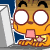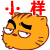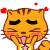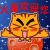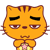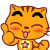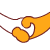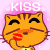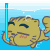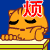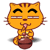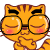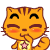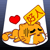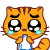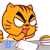12